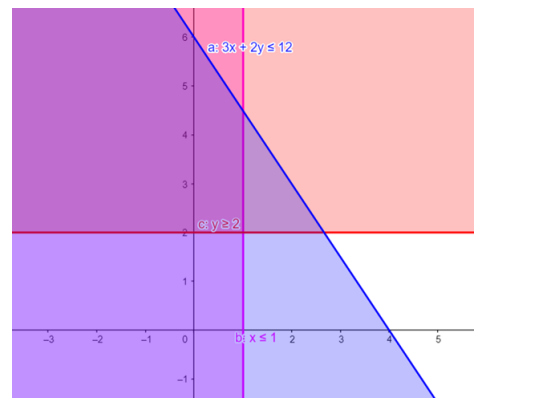# Solve the given inequalities

Question:

Solve the given inequalities $3 x+2 y \leq 12, x \leq 1, y \geq 2$ graphically in two - dimensional plane.

Solution:

The graphical representation of $3 x+2 y \leq 12, x \leq 1, y \geq 2$ is given by common region in the figure below.

$3 x+2 y \leq 12 \ldots \ldots$ (1)

$x \leq 1 \ldots \ldots .(2)$

$y \geq 2 \ldots \ldots$ (3)

Inequality $(1)$ represents the region below line $3 x+2 y=12$ (including the line $3 x+2 y=12$ ).

Inequality (2) represents the region behind line $x=1$ (including the line $\mathrm{x}=1$ ).

Inequality $(3)$ represents the region above line $y=2$ (including the line $y=2$ ).

Therefore, every point in the common shaded region including the points on the respective lines represents the solution for the given inequalities.

This can be represented as follows,